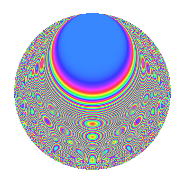# Properties

 Label 1089.2.fLevel 1089 Weight 2 Character orbit f Rep. character $$\chi_{1089}(487,\cdot)$$ Character field $$\Q(\zeta_{5})$$ Dimension 164 Sturm bound 264

# Related objects

## Defining parameters

 Level: $$N$$ = $$1089 = 3^{2} \cdot 11^{2}$$ Weight: $$k$$ = $$2$$ Character orbit: $$[\chi]$$ = 1089.f (of order $$5$$ and degree $$4$$) Character conductor: $$\operatorname{cond}(\chi)$$ = $$11$$ Character field: $$\Q(\zeta_{5})$$ Sturm bound: $$264$$

## Dimensions

The following table gives the dimensions of various subspaces of $$M_{2}(1089, [\chi])$$.

Total New Old
Modular forms 624 196 428
Cusp forms 432 164 268
Eisenstein series 192 32 160

## Trace form

 $$164q - 4q^{2} - 36q^{4} - 3q^{5} + 8q^{7} + 8q^{8} + O(q^{10})$$ $$164q - 4q^{2} - 36q^{4} - 3q^{5} + 8q^{7} + 8q^{8} + 24q^{10} + 8q^{13} - 2q^{14} - 46q^{16} + 14q^{17} + 8q^{19} + 6q^{20} + 32q^{23} - 36q^{25} - 16q^{26} - 24q^{28} - 4q^{29} - 13q^{31} - 48q^{32} - 32q^{34} + 13q^{37} - 2q^{38} + 12q^{40} + 20q^{41} + 16q^{43} + 32q^{46} + 14q^{47} + 27q^{49} + 18q^{50} - 16q^{52} + 18q^{53} + 60q^{56} + 10q^{58} - 35q^{59} - 24q^{61} - 28q^{62} - 58q^{64} - 28q^{65} - 52q^{67} - 28q^{68} + 16q^{70} - 17q^{71} - 44q^{73} - 30q^{74} - 8q^{76} - 24q^{79} - 36q^{80} + 6q^{82} + 34q^{83} - 44q^{85} + 48q^{89} - 44q^{91} - 12q^{92} + 8q^{94} + 30q^{95} + 43q^{97} + 40q^{98} + O(q^{100})$$

## Decomposition of $$S_{2}^{\mathrm{new}}(1089, [\chi])$$ into newform subspaces

The newforms in this space have not yet been added to the LMFDB.

## Decomposition of $$S_{2}^{\mathrm{old}}(1089, [\chi])$$ into lower level spaces

$$S_{2}^{\mathrm{old}}(1089, [\chi]) \cong$$ $$S_{2}^{\mathrm{new}}(33, [\chi])$$$$^{\oplus 4}$$$$\oplus$$$$S_{2}^{\mathrm{new}}(99, [\chi])$$$$^{\oplus 2}$$$$\oplus$$$$S_{2}^{\mathrm{new}}(121, [\chi])$$$$^{\oplus 3}$$$$\oplus$$$$S_{2}^{\mathrm{new}}(363, [\chi])$$$$^{\oplus 2}$$

## Hecke Characteristic Polynomials

There are no characteristic polynomials of Hecke operators in the database